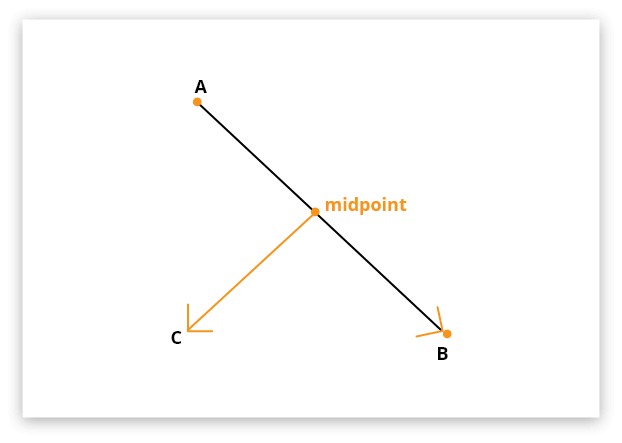# Midpoint Calculator

Enter the values of x1, y1, x2, and y2 in the given input boxes. Use the Calculate button to find midpoint between two points and the Reset button to enter new values

Show Steps## Midpoint formula

Midpoint formula can be expressed as:

(x1+x2)/2 , (y1+y2)/2

Where x and y represent the points in the Cartesian coordinate plane.

Midpoint calculator is an awesome utility to find the midpoint of the segment with the given endpoints. We will be discussing how to find the midpoint of a line segment, the formula of the midpoint, and much more in this space.

## What is the midpoint?

A midpoint is a point on a line segment that divides it into two equal parts. It is also known as the halfway point of a line segment.

Simply stated, a point at or near the centre or middle is termed as the midpoint. It is also defined as a point, line or plane that bisects a line.## How to find the midpoint of a line?

Follow these steps to find the midpoint without calculator.

Example:
Find the coordinates of the midpoint of the line joining (1, -3), (-5, 7).

Step 1: Identify and write down the values.

x1 = 1, y1 = -3
x2 = -5, y2 = 7

Step 2: Apply formula of the midpoint.

(x1+x2)/2, (y1+y2)/2
= ((1 + (-5))/2), (-3 +7)/2
= (-4/2, 4/2)
-2, 2

Besides the manual method to find a midpoint, a midpoint finder could ease the whole process if you are in a rush.

## FAQs

### How do you find the middle point between the two locations?

The middle point between the two locations can be found using the below equation:

M = (x1+x2)/2, (y1+y2)/2

Place the coordinates of both locations in the equation to get the midpoint.

### How do you find the endpoint given the midpoint?

An endpoint can be calculated using the below equation:

(x2, y2) = (2m1 – x1, 2m2 – y1)

Where, x2, y2 are the unknown endpoints, m1, m2 are the midpoints, and x1, x2 are the given endpoints. Moreover, you can also find endpoints using an endpoint calculator.

### How do you find the midpoint in stats?

The midpoint in stats can be calculated using the below formula:

Midpoint = (Lower class limit + Upper class limit)/2

1. Stapel, E. (2021). The Midpoint Formula | Purplemath.
2. Midpoint formula | Analytic geometry (article) | Khan Academy.